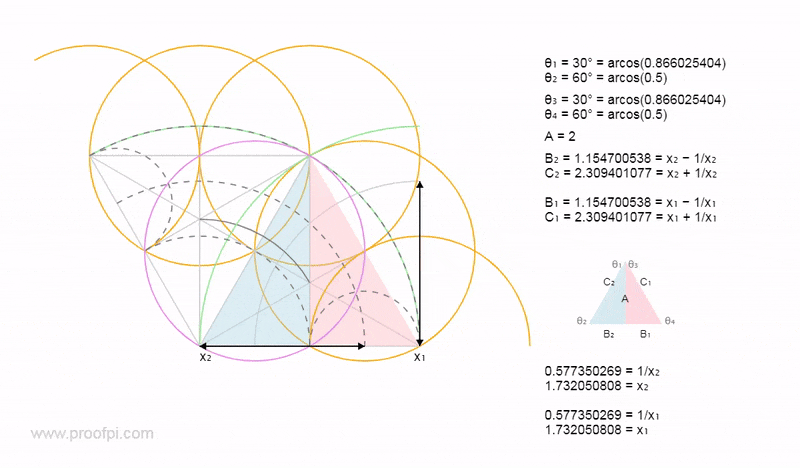A detailed look at the mathematics of the giza plateau, including the Sphinx and the three pyramids of the pharaohs Khufu, Khafra, and Menkaura.

Currently in the process of building my mathematical geometry engine. V3.0## The search for proof

I post all of my questions on the website (math.stackexchange), this allows over 10,000 mathematicians from all over the world to critique my work.

Some interesting things about the amazing mathematical cosmic identity.After 3 years I think I am finally getting somewhere. Some of my mathematical art.## The Universal Diagram

The following interactive diagram unites the triangle, square and the circle. Please use the following versions of (4/π+π/4), or try a cosmic number.

a) 2.0586377081326109957667309528 (calculator Pi)
b) 2.0578869977020399139902399165 (rootwo Pi)
c) 2.0581710272714922503219810475 (golden Pi)

Universal Maths
= (X) ≥ 1
= (1/X) ≤ 1

= B = (X - 1/X)
= C = (X + 1/X)

show values/hide equations?

Yes, I know that in 1882 Lindemann proved that π (3.141) is transcendental, but nobody has ever proved that π = 3.141. The way the value is currenlty calculated is by using a method of integration, and as you can see here, I believe at best it is a very close approximation.

## The search for symmetry

Because the circle and the square are both symmetrical, we would expect pi/the 'squared circle' to have perfect symmetry.

Below we can see the symmetry of the tangents to the arc.